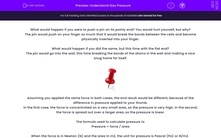# Understand Gas Pressure

In this worksheet, students will revise the concept of gas pressure in relation to the Particle Model.Key stage:  KS 3

Curriculum topic:   Physics: Matter

Curriculum subtopic:   Particle Model

Popular topics:   Physics worksheets

Difficulty level:#### Worksheet Overview

What would happen if you were to push a pin on its pointy end? You would hurt yourself, but why?

The pin would push on your finger so much that it would break the bonds between the cells and become physically inserted into your finger.

What would happen if you did the same, but this time with the flat end?

The pin would go into the wall, this time breaking the bonds of the atoms in the wall and making a nice snug home for itself.Assuming you applied the same force in both cases, the end result would be different because of the difference in pressure applied to your thumb.

In the first case, the force is concentrated on a very small area, so the pressure is very high. In the second, the force is spread out over a larger area, so the pressure is lower.

The formula used to calculate pressure is:

Pressure = force / area

When the force is in Newton (N) and the area in m2, the unit for pressure is Pascal (Pa) or N/m2.

If the area is in cm2, then the pressure is measured in N/cm2.

This means that if you increase the force then the pressure goes up.

Pressure = force divided by area; this means that pressure is inversely proportional to pressure.

This means that as area increases, pressure decreases.

Use your imagination to visualise how gas particles collide into the walls of the containers in the diagram below. Each time they hit the walls of the container, they push them a little bit. This is where our force comes from in the equation: pressure = force/area.

In this example, the second container has a smaller volume, but the number of particles is the same, so the particles collide more. Remember, every time there is a collision, there is a small force – so if there are more collisions, the total force increases.Boyle's Law is used to calculate how much pressure changes when the volume of a gas changes, provided the temperature and mass of the gas stay the same:

P1 × V1 = P2 × V2

P1 and V1 is the pressure and volume at the start, and P2 and V2 are the final pressure and volume of the gas.

If you rapidly change the volume of the container, then you will change the pressure as well as there will be more collisions in a smaller area – both of these are conditions for higher pressure.

### What is EdPlace?

We're your National Curriculum aligned online education content provider helping each child succeed in English, maths and science from year 1 to GCSE. With an EdPlace account you’ll be able to track and measure progress, helping each child achieve their best. We build confidence and attainment by personalising each child’s learning at a level that suits them.

Get started#### Popular Science topics

••••